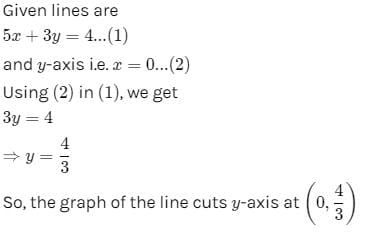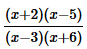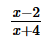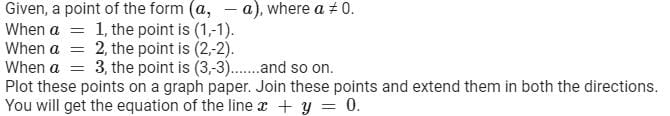Test: Linear Equations In Two Variables - 1

# Test: Linear Equations In Two Variables - 1

Test Description

## 25 Questions MCQ Test NCERT Mathematics for CAT Preparation | Test: Linear Equations In Two Variables - 1

Test: Linear Equations In Two Variables - 1 for Class 9 2022 is part of NCERT Mathematics for CAT Preparation preparation. The Test: Linear Equations In Two Variables - 1 questions and answers have been prepared according to the Class 9 exam syllabus.The Test: Linear Equations In Two Variables - 1 MCQs are made for Class 9 2022 Exam. Find important definitions, questions, notes, meanings, examples, exercises, MCQs and online tests for Test: Linear Equations In Two Variables - 1 below.
Solutions of Test: Linear Equations In Two Variables - 1 questions in English are available as part of our NCERT Mathematics for CAT Preparation for Class 9 & Test: Linear Equations In Two Variables - 1 solutions in Hindi for NCERT Mathematics for CAT Preparation course. Download more important topics, notes, lectures and mock test series for Class 9 Exam by signing up for free. Attempt Test: Linear Equations In Two Variables - 1 | 25 questions in 25 minutes | Mock test for Class 9 preparation | Free important questions MCQ to study NCERT Mathematics for CAT Preparation for Class 9 Exam | Download free PDF with solutions
 1 Crore+ students have signed up on EduRev. Have you?
Test: Linear Equations In Two Variables - 1 - Question 1

### x = 2, y = – 1 is a solution of the line equal to :

Test: Linear Equations In Two Variables - 1 - Question 2

### The straight line passing through the points (0, 0), (–1, 1) and (1, – 1) has the equation :

Test: Linear Equations In Two Variables - 1 - Question 3

### The graph of the equation 2x – 3 = 3x – 5 is parallel to :

Test: Linear Equations In Two Variables - 1 - Question 4

The straight line 2x – 5y = 0 passes through the point :

Test: Linear Equations In Two Variables - 1 - Question 5

The graph of the lines x + y = 7 and x – y = 3 meet at the point :

Test: Linear Equations In Two Variables - 1 - Question 6

The graph of the equation y = x2 is :

Detailed Solution for Test: Linear Equations In Two Variables - 1 - Question 6

In the graph of y = x2, the point (0, 0) is called the vertex. The vertex is the minimum point in a parabola that opens upward. In a parabola that opens downward, the vertex is the maximum point. We can graph a parabola with a different vertex.

Test: Linear Equations In Two Variables - 1 - Question 7

Which of the following equations is not linear equation :

Test: Linear Equations In Two Variables - 1 - Question 8

If x = 1,y = 1 is a solution of equation 9ax + 12ay = 63 then, the value of a is :

Test: Linear Equations In Two Variables - 1 - Question 9

The graph of the line 5x + 3y = 4 cuts y-axis at the point :

Detailed Solution for Test: Linear Equations In Two Variables - 1 - Question 9Test: Linear Equations In Two Variables - 1 - Question 10

A linear equation in two variables has maximum :

Test: Linear Equations In Two Variables - 1 - Question 11

The equation=has

Test: Linear Equations In Two Variables - 1 - Question 12

If x = a, y = b is the solution of the pair of equation x-y = 2 and x+y = 4 then what will be value of a and b

Test: Linear Equations In Two Variables - 1 - Question 13

The solution of the equation x + y = 3, 3x – 2y = 4 is :

Test: Linear Equations In Two Variables - 1 - Question 14

The value of x satisfying the equation x2 + p2 = (q – x)2 is :

Detailed Solution for Test: Linear Equations In Two Variables - 1 - Question 14

Solution :

The correct option is Option B.

n² + p² = (q + n)²

n² + p² = q² - 2qn + n²

Eliminating n² from both sides,

p² = q² - 2qn

n = q² - p² / 2q

Test: Linear Equations In Two Variables - 1 - Question 15

The sum of two digits and the number formed by interchanging its digit is 110. If ten is subtracted from the first number, the new number is 4 more than 5 times of the sum of the digits in the first number. Find the first number.

Detailed Solution for Test: Linear Equations In Two Variables - 1 - Question 15

let the unit place digit be x and tens place digit be y

then the two-digit number will be  10y + x

and the number formed by interchanging the unit place and tens place digits will be 10x + y

according to the first condition given in the qs i.e, the sum of two numbers is 110 that  is

10y + x + 10x + y = 110

=> 11x + 11y = 110

divide the above equation by 11 we get

x + y = 10

x = 10 - y ....(i)

now according to the second equation,

if 10 is subtracted from the first number i.e, the new number is  10y + x - 10

given that the new number is 4 more than 5 time the sum of its digits in the first number i.e

the sum of its digits in the first number is  x + y,  now 5 times of its, 5(x + y), and now 4 more that is, 4 + 5(x + y)

therefore new number = 4 + 5(x + y)

10y + x - 10 = 4 +5(x + y)

10y - 5y + x = 4 +10 +5x

5y = 14 + 4x.....(ii)

substitute the value of x from eq(i) to eq (ii)

we  get , 5y = 14 + 4(10 - y)

5y = 14 + 40 - 4y

y = 6

and from eq(i)

x = 4

then the first number 10y + x = 10x6 + 4 = 64

first number is 64.

Test: Linear Equations In Two Variables - 1 - Question 16

x = 0 is the equation of

Test: Linear Equations In Two Variables - 1 - Question 17

y = 0 is the equation of

Test: Linear Equations In Two Variables - 1 - Question 18

x – 4 is the equation of

Test: Linear Equations In Two Variables - 1 - Question 19

y + 7 is the equation of

Test: Linear Equations In Two Variables - 1 - Question 20

The point of the form (a, -a), where  a ≠ 0, lies on

Detailed Solution for Test: Linear Equations In Two Variables - 1 - Question 20Test: Linear Equations In Two Variables - 1 - Question 21

The point of the form (a, -a), where a , 0 lies on

Test: Linear Equations In Two Variables - 1 - Question 22

The point of the form (-a, a), where a , 0 lies on

Test: Linear Equations In Two Variables - 1 - Question 23

The linear equation 2x + 3y = 6 has

Test: Linear Equations In Two Variables - 1 - Question 24

The graph of the linear equation 2x – 3y = 6, cuts the y-axis at the point

Test: Linear Equations In Two Variables - 1 - Question 25

The graph of the linear equation 3x – 2y = 6, cuts the x-axis at the point

## NCERT Mathematics for CAT Preparation

276 docs|149 tests
 Use Code STAYHOME200 and get INR 200 additional OFF Use Coupon Code
Information about Test: Linear Equations In Two Variables - 1 Page
In this test you can find the Exam questions for Test: Linear Equations In Two Variables - 1 solved & explained in the simplest way possible. Besides giving Questions and answers for Test: Linear Equations In Two Variables - 1, EduRev gives you an ample number of Online tests for practice

## NCERT Mathematics for CAT Preparation

276 docs|149 tests